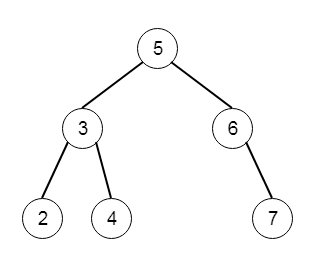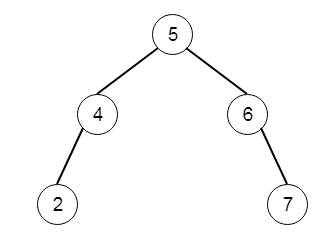# Delete Node in a BST in C++

Suppose we have a binary search tree. We will take one key k, and we have to delete the given key k from the BST, and return the updated BST. So if the tree is like −And the key k = 3, then the output tree will be −To solve this, we will follow these steps −

• Define a method called deleteRoot() to delete the root node, this will work as follows

• if root is null, then return null

• if root has no right subtree, then return left of root

• x := inorder successor of root

• set left of x as left := left of root

• return right of root

• The delete method will be like

• if root is null or value of root is key, then return deleteRoot(root)

• curr := root

• Create one infinite loop, and execute the following

• x := value of curr node

• if key < x, then

• if left of curr = null or value of left of curr = key, then

• left of curr := deleteRoot(left of curr) and come out from the loop.

• curr := left of curr

• otherwise

• if right of curr = null or value of right of curr = key, then

• right of curr := deleteRoot(right of curr) and come out from loop.

• curr := right of curr

• return root

Let us see the following implementation to get better understanding −

## Example

Live Demo

#include <bits/stdc++.h>
using namespace std;
class TreeNode{
public:
int val;
TreeNode *left, *right;
TreeNode(int data){
val = data;
left = NULL;
right = NULL;
}
};
void insert(TreeNode **root, int val){
queue<TreeNode*> q;
q.push(*root);
while(q.size()){
TreeNode *temp = q.front();
q.pop();
if(!temp->left){
if(val != NULL)
temp->left = new TreeNode(val);
else
temp->left = new TreeNode(0);
return;
} else {
q.push(temp->left);
}
if(!temp->right){
if(val != NULL)
temp->right = new TreeNode(val);
else
temp->right = new TreeNode(0);
return;
} else {
q.push(temp->right);
}
}
}
TreeNode *make_tree(vector<int> v){
TreeNode *root = new TreeNode(v);
for(int i = 1; i<v.size(); i++){
insert(&root, v[i]);
}
return root;
}
void tree_level_trav(TreeNode*root){
if (root == NULL) return;
cout << "[";
queue<TreeNode *> q;
TreeNode *curr;
q.push(root);
q.push(NULL);
while (q.size() > 1) {
curr = q.front();
q.pop();
if (curr == NULL){
q.push(NULL);
}
else {
if(curr->left)
q.push(curr->left);
if(curr->right)
q.push(curr->right);
if(curr == NULL || curr->val == 0){
cout << "null" << ", ";
} else {
cout << curr->val << ", ";
}
}
}
cout << "]"<<endl;
}
class Solution {
public:
TreeNode* deleteNode(TreeNode* root, int key) {
if(root == NULL || root->val == key) return deleteRoot(root);
TreeNode* curr = root;
while(1) {
int x = curr->val;
if(key < x){
if(curr->left == NULL || curr->left->val == key){
curr->left = deleteRoot(curr->left);
break;
}
curr = curr->left;
} else {
if(curr->right == NULL || curr->right->val == key){
curr->right = deleteRoot(curr->right);
break;
}
curr = curr->right;
}
}
return root;
}
TreeNode* deleteRoot(TreeNode* root){
if(!root || root->val == 0)return NULL;
if(root->right == NULL) return root->left;
TreeNode* x = root->right;
while(x->left)x = x->left;
x->left = root->left;
return root->right;
}
};
main(){
vector<int> v = {5,3,6,2,4,NULL,7};
TreeNode *root = make_tree(v);
Solution ob;
tree_level_trav(ob.deleteNode(root, 3));
}

## Input

[5,3,6,2,4,null,7]
3

## Output

[5, 4, 6, 2, null, 7, ]

Updated on: 30-Apr-2020

3K+ Views